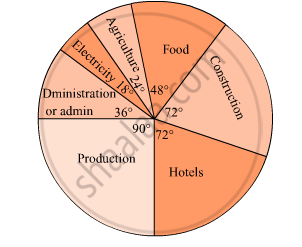# The Pie Diagram in Figure Shows the Proportions of Different Workers in a Town. Answer the Following Question with Its Help. How Many Workers Are Working in the Administration ? - Algebra

Short Note

The pie diagram in figure shows the proportions of different workers in a town. Answer the following question with its help.

How many workers are working in the administration ?#### Solution

Number of workers working in the administration
$= \frac{\text{ central angle for administration }}{360^\circ} \times 10000$

$= \frac{36^\circ}{360^\circ} \times 10000$

= 1000

Concept: Concept of Pie Graph (Or a Circle-graph)
Is there an error in this question or solution?

#### APPEARS IN

Balbharati Mathematics 1 Algebra 10th Standard SSC Maharashtra State Board
Chapter 6 Statistics
Practice Set 6.6 | Q 5.2 | Page 164